# Mensuration II (Important results)

The following results are very important to solve various mensuration problems.

1.  The largest possible sphere that can be chiseled out from a cube of side "a" cm.Diagonal of the sphere is a, so radius = a/2.
Remaining empty space in the cube = ${a^3} - \displaystyle\frac{{\pi {a^3}}}{6}$

2. The largest possible cube that can be chiseled out from a sphere of radius "a" cmHere OA = radius of the sphere. So diameter of the sphere = 2a.
Let the side of the square = $x$, then the diagonal of the cube = $\sqrt 3 x$
$\Rightarrow \sqrt 3 x = 2a$ $\Rightarrow x = \dfrac{{2a}}{{\sqrt 3 }}$
Therefore side of the square = $\displaystyle\frac{{2a}}{{\sqrt 3 }}$

3.  The largest possible cube that can be chiseled out from a hemisphere of radius 'a' cm.Sol:Given, the radius of the hemi sphere AC = $a$.  Let the side of the cube is $x$.
From the above diagram, $B{E^2} + E{D^2} = B{D^2}$
$\Rightarrow {x^2} + {x^2} = B{D^2}$
$\Rightarrow BD = \sqrt 2 x$
$\Rightarrow BC = \dfrac{{\sqrt 2 x}}{2} = \dfrac{x}{{\sqrt 2 }}$
From $\Delta$ABC, $A{C^2} = A{B^2} + B{C^2}$
$\Rightarrow {a^2} = {x^2} + {\left( {\dfrac{x}{{\sqrt 2 }}} \right)^2}$
$\Rightarrow {a^2} = \dfrac{{3{x^2}}}{2}$
$\Rightarrow x = \sqrt {\dfrac{2}{3}} a$
The edge of the cube = $a\sqrt {\displaystyle\frac{2}{3}}$

4.  The largest square that can be inscribed in a right angled triangle ABC when one of its vertices coincide with the vertex of right of the triangle.Solution: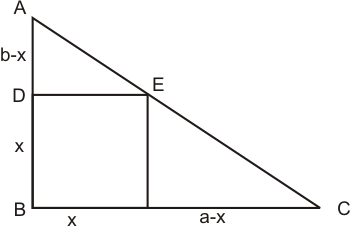let the side of the square = $x$
DE // BC, therefore, $\Delta$ADE and $\Delta$ABC are similar.
$\Rightarrow \dfrac{{AD}}{{AB}} = \dfrac{{DE}}{{BC}}$
$\Rightarrow \dfrac{{b - x}}{b} = \dfrac{x}{a}$
$\Rightarrow 1 - \dfrac{x}{b} = \dfrac{x}{a}$
$\Rightarrow 1 = \dfrac{x}{b} + \dfrac{x}{a}$
$\Rightarrow 1 = x\left( {\dfrac{{a + b}}{{ab}}} \right)$
$\Rightarrow x = \left( {\dfrac{{ab}}{{a + b}}} \right)$
Side of the square = $\dfrac{{ab}}{{a + b}}$ and area of the square = ${\left( {\dfrac{{ab}}{{a + b}}} \right)^2}$

5.  The largest square that can be inscribed in a right angled triangle ABC when one of its vertices lies on the hypotenuse of the triangleSolution 1: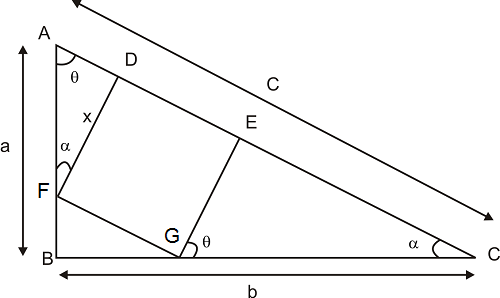From the above diagram,  $\Delta$ABC and $\Delta$AFD are similar.
$\Rightarrow Tan\alpha = \dfrac{{AD}}{{FD}} = \dfrac{{AB}}{{BC}}$
$\Rightarrow \dfrac{{AD}}{x} = \dfrac{a}{b}$
$\Rightarrow AD = \dfrac{{xa}}{b}$ - - - - - (1)
Also, $\Delta$ABC and $\Delta$EGC are similar.
$\Rightarrow Tan\alpha = \dfrac{{GE}}{{EC}} = \dfrac{a}{b}$
$\Rightarrow \dfrac{x}{{EC}} = \dfrac{a}{b}$
$\Rightarrow EC = \dfrac{{xb}}{a}$ - - - - - (2)
We know that c= AD + x + EC
$\Rightarrow c = \dfrac{{xa}}{b} + x + \dfrac{{xb}}{a}$
$\Rightarrow c = x\left( {\dfrac{a}{b} + 1 + \dfrac{b}{a}} \right)$
$\Rightarrow c = x\left( {\dfrac{{{a^2} + ab + {b^2}}}{{ab}}} \right)$
$\Rightarrow x = \left( {\dfrac{{abc}}{{{a^2} + ab + {b^2}}}} \right)$
Side of the square = ${\displaystyle\frac{{abc}}{{{a^2} + {b^2} + ab}}}$

Solution 2: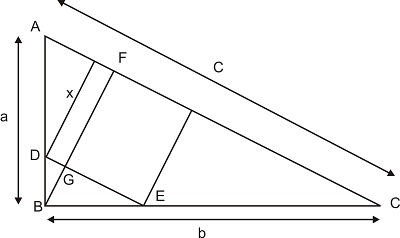From the above diagram, drop a perpendicular to AC from vertex B.
Area of $\Delta$ABC = $\dfrac{1}{2} \times a \times b = \dfrac{1}{2} \times BF \times c$
$\therefore BF = \dfrac{{ab}}{c}$ - - - - - (1)
Now $\Delta$BDE and $\Delta$ABC are similar.
$\Rightarrow \dfrac{{BG}}{{BF}} = \dfrac{{DE}}{{AC}}$
$\Rightarrow \dfrac{{\dfrac{{ab}}{c} - x}}{{\dfrac{{ab}}{c}}} = \dfrac{x}{c}$
$\Rightarrow 1 - \dfrac{{xc}}{{ab}} = \dfrac{x}{c}$
$\Rightarrow 1 = \dfrac{{xc}}{{ab}} + \dfrac{x}{c}$
$\Rightarrow 1 = x\left( {\dfrac{c}{{ab}} + \dfrac{1}{c}} \right)$
$\Rightarrow 1 = x\left( {\dfrac{{{c^2} + ab}}{{abc}}} \right)$
$\Rightarrow x = \dfrac{{abc}}{{{c^2} + ab}}$ = $\dfrac{{abc}}{{{a^2} + {b^2} + ab}}$

6.  The largest square that can be inscribed in a semi circle of radius 'r' units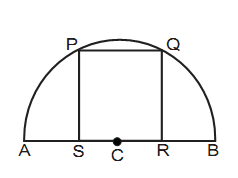Area of the square = $\displaystyle\frac{3}{5}{r^2}$

7.  The largest square that can be inscribed in a quadrant of radius 'r' cm.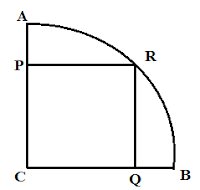Side of the square = $\displaystyle\frac{r}{{\sqrt 2 }}$, and area of the square = $\displaystyle\frac{{{r^2}}}{2}$

8.  The largest circle that can be inscribed in the semi circle of radius 'r' cm isInscribed circle area = $\displaystyle\frac{{\pi {r^2}}}{4}$
(Rememeber: Inscribed circle area is half of the semi circle area)

9.  The largest circle that can be inscribed in a quadrant of radius 'r' cm is

Area of the circle = $\displaystyle\frac{{\pi {r^2}}}{{3 + 2\sqrt 2 }}$

Level - 2:

10. The largest cube that can be chiseled out from a cone of height 'h' cm and radius of 'r' cm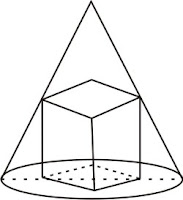Solution:Let the side of the square = $x$
DHE is a right angle triangle.   Therefore, $D{E^2} = D{H^2} + H{E^2}$
$\Rightarrow DE = \sqrt {{x^2} + {x^2}} = \sqrt 2 x$
From the diagram, $\Delta ADE$ and $\Delta ABC$ are similar.
$\Rightarrow \dfrac{{AF}}{{AG}} = \dfrac{{DE}}{{BC}}$
$\Rightarrow \dfrac{{h - x}}{h} = \dfrac{{\sqrt 2 x}}{{2r}}$
$\Rightarrow 1 - \dfrac{x}{h} = \dfrac{x}{{\sqrt 2 r}}$
$\Rightarrow \dfrac{x}{{\sqrt 2 r}} + \dfrac{x}{h} = 1$
$\Rightarrow x\left( {\dfrac{1}{{\sqrt 2 r}} + \dfrac{1}{h}} \right) = 1$
$\Rightarrow x\left( {\dfrac{{h + \sqrt 2 r}}{{\sqrt 2 rh}}} \right) = 1$
$\Rightarrow x = \dfrac{{\sqrt 2 rh}}{{h + \sqrt 2 r}}$
Square side = $\dfrac{{\sqrt 2 rh}}{{h + \sqrt 2 r}}$

11.  Find the maximum volume of cylinder that can be made out of a cone of radius 'r' and height 'h'Sol:
Let the radius of the cylinder = $x$ and height = $y$.  We have to maximize the volume of the cylinder V = $\pi {x^2}y$.  To maximize the volume we have to change one of the given variable in the equation into "r"and "h" and differentiate the equation with respect to the other variable.From the above, $\Delta ADE$ and $\Delta ABC$ are similar.
So, $\Rightarrow \dfrac{{h - y}}{h} = \dfrac{{2x}}{{2r}}$ - - - - - (1)
$\Rightarrow h - y = \dfrac{{xh}}{r}$
$\Rightarrow h - \dfrac{{xh}}{r} = y$
Substituting the above result in the volume we get,
$V = \pi {x^2}\left( {h - \dfrac{{hx}}{r}} \right)$
$V = \pi h{x^2} - \dfrac{{\pi h{x^3}}}{r}$
Differentiation the above equation w.r.t x,
${V^1}(x) = 2\pi hx - \dfrac{{3\pi h{x^2}}}{r}$
By equating the above equation to zero, we can find the value of x where the above equation becomes maximum.
$2\pi hx - \dfrac{{3\pi h{x^2}}}{r}$ = 0
$\Rightarrow 2\pi hx\left( {2 - \dfrac{{3x}}{r}} \right) = 0$
h, x cannot be zero. So ${2 - \dfrac{{3x}}{r}}$ = 0
$\Rightarrow x = \dfrac{{2r}}{3}$ - - - - - (2)
Substituting the above result in equation (1),
$\Rightarrow \dfrac{{h - y}}{h} = \dfrac{{2\left( {\dfrac{{2r}}{3}} \right)}}{{2r}}$
$\Rightarrow \dfrac{{h - y}}{h} = \dfrac{2}{3}$
$\Rightarrow h - y = \dfrac{2}{3}h$
$\Rightarrow y = \dfrac{h}{3}$ - - - - - (3)
Therefore, maximum volume of the cylinder = $\pi {\left( {\dfrac{{2r}}{3}} \right)^2}\left( {\dfrac{h}{3}} \right)$
$\Rightarrow \dfrac{4}{{27}}\pi {r^2}h$

12. Find the maximum volume of sphere that can be inscribed in a cone.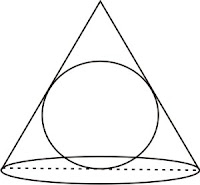Sol:Let the cone height = $h$ and radius = $r$.  Also let the radius of the sphere inside the cone = $x$
We have to maximize the volume of the sphere = $V = \dfrac{4}{3}\pi {x^3}$
From the above diagram, $\Delta AFC$ and $\Delta ADE$ are similar.  ($\because$ AAA rule)
Therefore, $\dfrac{{CF}}{{DE}} = \dfrac{{AC}}{{AD}}$
From Pythagoras rule, $AC = \sqrt {A{F^2} + F{C^2}} = \sqrt {{h^2} + {r^2}}$
Now, $\dfrac{r}{x} = \dfrac{{\sqrt {{r^2} + {h^2}} }}{{h - x}}$
$\Rightarrow rh - rx = x\sqrt {{r^2} + {h^2}}$
$\Rightarrow rh = rx + x\sqrt {{r^2} + {h^2}}$
$\Rightarrow rh = x\left( {r + \sqrt {{r^2} + {h^2}} } \right)$
$\Rightarrow x = \dfrac{{rh}}{{r + \sqrt {{r^2} + {h^2}} }}$

13. Find the maximum volume of cone that can be inscribed in a sphere.Sol: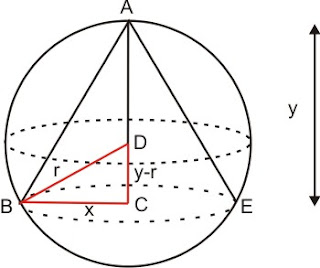Let the radius of the sphere = $r$.
Let the radius of the cone = $x$, and height = $y$
We have to maximize the volume of the cone = V = $\dfrac{1}{3}\pi {x^2}y$ - - - - - (1)
From $\Delta$BCD, $B{D^2} = B{C^2} + C{D^2}$
$\Rightarrow {r^2} = {x^2} + {\left( {y - r} \right)^2}$
$\Rightarrow {r^2} = {x^2} + {y^2} + {r^2} - 2yr$
$\Rightarrow {x^2} = 2yr - {y^2}$ - - - - (2)
Substituting the above result in equation (1), and differentiating w.r.t. y, and equating to zero,
V= $\dfrac{1}{3}\pi \left( {2yr - {y^2}} \right)y$
${V^1}(y) = \dfrac{1}{3}\pi \left( {4yr - 3{y^2}} \right)$ = 0
$\Rightarrow y = \dfrac{{4r}}{3}$
Now CD = $\dfrac{{4r}}{3} - r = \dfrac{r}{3}$
From $\Delta$BCD,
${r^2} = {x^2} + {\left( {\dfrac{r}{3}} \right)^2}$
$\Rightarrow x = \sqrt {\dfrac{{8{r^2}}}{9}} = \dfrac{{2\sqrt 2 r}}{3}$ - - - - (3)
Volume of the cone = $\dfrac{1}{3}\pi {x^2}y$ = $\dfrac{1}{3}\pi {\left( {\dfrac{{2\sqrt 2 r}}{3}} \right)^2}\dfrac{{4r}}{3} = \dfrac{{32}}{{81}}\pi {r^3}$